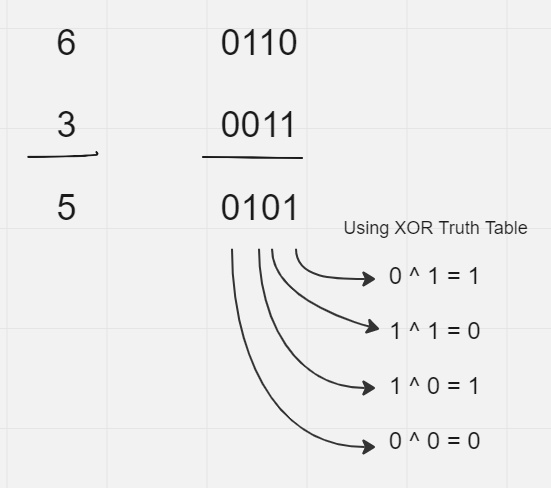# Python Bitwise XOR Operator and its Uses

Operators are used in a programming language to perform operations on values at the level of individual bits. Some examples of bitwise operators are AND, OR, not, and XOR. Since all these operators work on individual bits, it is called bitwise operators.

In Python, the bitwise operator is also called the binary operator and it is used to perform various bitwise calculations on integers. The operators first convert the integers into binary format and then perform calculation bit by bit.

## XOR Operator in Python

In Python, the XOR operator is known as the “exclusive or” operator which is used to compare two binary numbers bitwise.

The XOR operator, if both the input values are the same it returns `0`, and if the input values are different it outputs `1`. We can also use the XOR operator on booleans.

The XOR operator is represented as `^` between two values (operands) to perform bitwise “exclusive or” calculations.

Syntax:

``a ^ b``

Here is a truth table of XOR operator.

a b a^b
0 0 0
0 1 1
1 0 1
1 1 0

Now, let’s see some examples to find XOR values of integers in Python.

## Find XOR value of two numbers in Python

Let’s find out the value of two integers using the XOR operators in this example. When we perform an XOR operation between two numbers it gives the output as an integer.

Example

``````a = 6 #0110
b = 3 #0011

print(a ^ b)``````

Output:

``5 #(0101)``

Here, first, the program converts the numbers into their binary format ie. for 6 (0110) and 3 (0011), and then using the XOR operator it calculates the output ie. 5 (0101) bit by bit.

Here is a demonstration to understand the calculation of the binary numbers using the XOR truth table.## Compare boolean using XOR in Python

We can use the XOR operator on boolean values too. In the case of a boolean, the `True` is treated as 1 and `False` is treated as 0.

When we compare two booleans in XOR the output is also a boolean value.

So the truth table for boolean would be like this.

a b a^b
False False False
False True True
True False True
True True False

Example of XOR boolean value in python

``````print(False ^ False)
print(False ^ True)
print(True ^ False)
print(True ^ True)``````

Output:

``````False
True
True
False``````

## Using Operator Module in Python

We can also use the in-built `operator` module to perform XOR operations in Python. It provides the `xor()` functions which can perform operations on integers and booleans.

Example:

``````import operator

print(operator.xor(6,3))
print(operator.xor(False,False))
print(operator.xor(False,True))``````

Output:

``````5
False
True``````

Here, we just have to pass the values as parameters to the `xor()` function and it returns the output according to the data type of the value (integer or boolean).

Conclusion : In this article, we have learned about the logical operator, the bitwise binary XOR operator, and how we can use it in python to compare and perform bitwise calculations on numbers and booleans.